# Workhorses

A team of colleagues done the work in 20 days. After adding 2 colleagues from the beginning the work was completed in 15 days. How many members has the colleague team?

Result

n =  6

#### Solution:

20n=15(n+2)

5n = 30

n = 6

Calculated by our simple equation calculator.

Leave us a comment of example and its solution (i.e. if it is still somewhat unclear...):Be the first to comment!#### To solve this example are needed these knowledge from mathematics:

Do you have a linear equation or system of equations and looking for its solution? Or do you have quadratic equation?

## Next similar examples:

1. Painters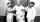One painter could paint a school for 7 days. The second one for 6 days. It was necessary to paint the school in two days. Next painter was invited and all worked together. How long would this painter painted the school itself?
2. Collection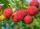John has collected half a bucket of raspberries in 45 minutes, Eva collects the whole bucket in an hour. How many minutes it would take to fill a bucket if the two children work together?
3. Sprayers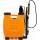The vineyard can be sprayed first sprayer in 5 hours and with the second in 8 hours. How long would it take if we use sprayers both at once?
4. Three peopleThree people start doing a work at a same time. The first worked only 2 hours. The second ended 3 hours before the end. On an individual basis, it would take the first time to do the work 10 hours, second 12 hours and 15 hours third. How many hours did it.
5. Tonic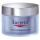One package tonic consume Mom 12 weeks and daughter for 14 weeks. 4 weeks they use the same cream together and then only daughter. How many weeks endured tonic to daughter then?
6. Two looms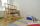On two different looms with different performance can be make required quantity of textile in 6 hours when both work together. On the first machine this quantity of the textile can make in 10 hours. How long should take make same quantity of textile on a s
7. Three figures - numbersThe sum of three numbers, if each is 10% larger than the previous one, is 662. Determine the figures.
8. Unknown numberIdentify unknown number which 1/5 is 40 greater than one tenth of that number.
9. EquationsSolve following system of equations: 6(x+7)+4(y-5)=12 2(x+y)-3(-2x+4y)=-44
10. ClassroomThere are eighty more girls in the class than boys. Boys are 40 percent and girls are 60 percent. How many are boys and how many girls?
11. Substitutionsolve equations by substitution: x+y= 11 y=5x-25
12. Grandfather and grandmotherThe old mother is 5 years younger than the old father. Together they are 153 years old. How many years has each of them?
13. Jane plantsJane plants flowers in the garden. If she planted 12 every hour instead of 9 flowers, she would finish with the job an hour earlier. How many flowers does she plant?
14. Fifth of the numberThe fifth of the number is by 24 less than that number. What is the number?
15. MushroomsEva and Jane collected 114 mushrooms together. Eve found twice as much as Jane. How many mushrooms found each of them?
16. Family parcelsIn father will he divided the land so that the older son had three bigger part than younger son. Later elder son gave 2.5 ha field to younger and they had both the same. Determine the area of family parcel.
17. Dining roomThe dining room has 11 tables (six and eight seats). In total there are 78 seats in the dining room. How many are six-and eight-seat tables?